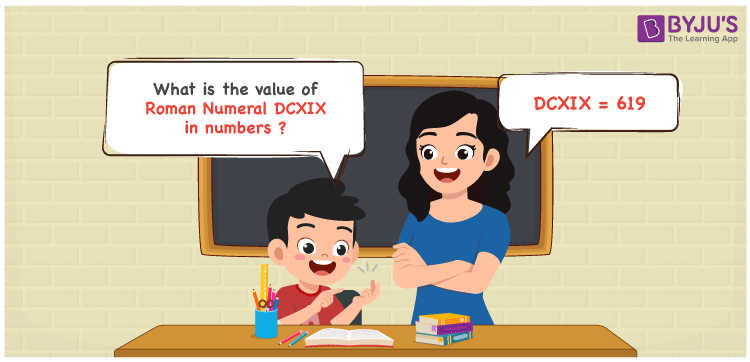Checkout JEE MAINS 2022 Question Paper Analysis : Checkout JEE MAINS 2022 Question Paper Analysis :

# DCXIX Roman Numerals

DCXIX Roman Numerals is 619. Understand the steps used to show the given roman numerals in numbers by going through this article. By using the error free materials given online, conversion steps can be memorized with ease based on the requirements. The experts focus to provide students with a clear knowledge of the roman numerals conversion for a strong base of the fundamentals. Hence, DCXIX can be written as 619 in numbers.

 Number Roman Numeral 619 DCXIX## How to Write DCXIX Roman Numerals in Numbers?

The expansion of DCXIX, transformation to numbers and the numeric form is provided here.

DCXIX = D + C + X + (X – I)

DCXIX = 500 + 100 + 10 + (10 – 1)

DCXIX = 619

## Video Lesson on Roman Numerals## Frequently Asked Questions on DCXIX Roman Numerals

### How 619 is written as DCXIX in roman numerals?

We know that;

600 in roman numerals is DC

19 in roman numerals is XIX

So 619 in roman numerals is DCXIX.

### Find 600 + 19.

We know that

600 + 19 = 619

Hence, 600 + 19 is 619 which is written as DCXIX.

### Determine the remainder if DCXIX is divided by IV.

We know that

DCXIX = 619

IV = 4

The remainder if DCXIX is divided by IV is III.